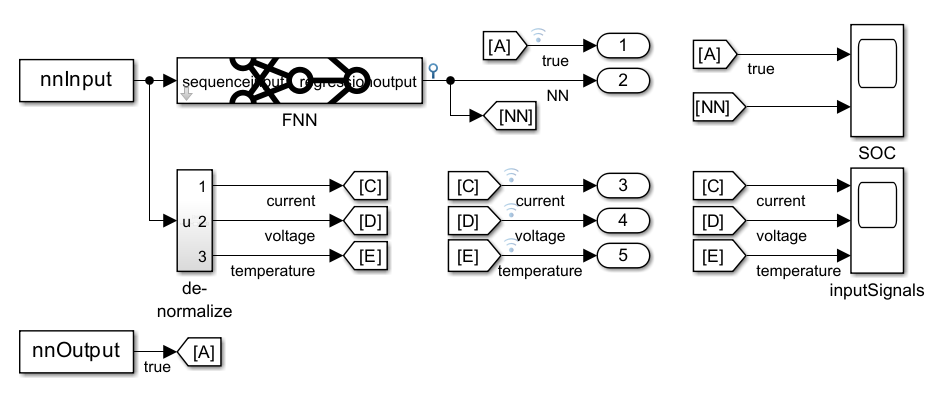# Improve Performance of Deep Learning Simulations in Simulink

This example shows how to use code generation to improve the performance of deep learning simulations in Simulink®.

By default, Simulink models simulate deep learning blocks using interpreted execution via the MATLAB® execution engine. You can choose to instead simulate deep leaning blocks using code generation, which supports optimized implementations for common computational functions used in deep neural networks. Using code generation instead of interpreted execution speeds up deep learning simulations, often requires no modification of the neural network or the Simulink model, and does not require additional product licenses such as MATLAB Coder™.

In this example, you improve the performance of the Simulink model from the Battery State of Charge Estimation in Simulink Using Deep Learning Network example by enabling code generation. This example uses the preprocessed data set `LG_HG2_Prepared_Dataset_McMasterUniversity_Jan_2020` from .

Open the Simulink model `BatterySOCSimulinkEstimation`.

```BatterySOCSimulinkEstimation_ini; model = "BatterySOCSimulinkEstimation"; open_system(model) activeConfigObj = getActiveConfigSet(model);```

The model uses a trained neural network `net` to predict the state of charge of a Li-ion battery for the input time-series data representing features of the battery such as the voltage, current, temperature, and the average voltage and current over the last 500 seconds.Display the layers of the trained neural network.

`net.Layers`
```ans = 8×1 Layer array with layers: 1 'sequenceinput' Sequence Input Sequence input with 5 dimensions 2 'fc_1' Fully Connected 55 fully connected layer 3 'layer' Tanh Hyperbolic tangent 4 'fc_2' Fully Connected 55 fully connected layer 5 'leakyrelu' Leaky ReLU Leaky ReLU with scale 0.3 6 'fc_3' Fully Connected 1 fully connected layer 7 'clippedrelu' Clipped ReLU Clipped ReLU with ceiling 1 8 'regressionoutput' Regression Output mean-squared-error with response 'Response' ```

For an example showing how to train the network, see Predict Battery State of Charge Using Deep Learning.

### Requirements for Improving Simulation Performance Using Code Generation

To enable execution using code generation in Simulink, perform these steps:

1. Install a supported compiler.

2. Install the add-on MATLAB Coder Interface for Deep Learning Libraries.

3. Ensure that all the layers in the deep neural network in your model support code generation.

4. Set the simulation target language to C++.

#### Install Supported C++ Compiler

On a Windows® operating system, a Microsoft® Visual C++® compiler is required. On a Linux® operating system, a GCC C/C++ compiler is required. The MinGW® compiler is not supported for this workflow. To view a list of compilers, see Supported and Compatible Compilers.

Verify that a supported C++ compiler is installed and selected.

```myCppCompiler = mex.getCompilerConfigurations('C++','Selected'); myCppCompiler.Name```
```ans = 'Microsoft Visual C++ 2019' ```

If you have multiple C++ compilers installed, display information for the installed compilers with the following command, then click one of the links.

```mex -setup cpp ```

#### Install MATLAB Coder Interface for Deep Learning Libraries

MATLAB Coder Interface for Deep Learning Libraries is a free add-on for MATLAB. With this add-on you can use the Intel Math Kernel Library for Deep Neural Networks (MKL-DNN). You do not need a MATLAB Coder license for this workflow.

To find and install add-ons, go to the Home tab and, in the Environment section, click the Add-Ons icon. The Add-On Explorer opens and displays the list of available add-ons. Using the search bar, find the MATLAB Coder Interface for Deep Learning Libraries add-on. Open the add-on and click the Install icon.

Verify that the add-on has properly installed using these commands.

```requiredAddOns = "MATLAB Coder Interface for Deep Learning Libraries"; matlab.addons.isAddonEnabled(requiredAddOns) ```

#### Verify Layers in Deep Neural Network Support Code Generation

Check that your network supports code generation using MKL-DNN.

`analyzeNetworkForCodegen(net,TargetLibrary="mkldnn")`
``` Supported _________ mkldnn "Yes" ```

To view a full list of deep learning layers that support code generation in Simulink, use the following command.

```supportedLayers = coder.getDeepLearningLayers ```

#### Set Simulation Target Language

The simulation target language defines the language of the generated code. By default, the simulation target language is C.

`get_param(activeConfigObj,"SimTargetLang")`
```ans = 'C' ```

To set the simulation target language to C++, use this command.

`set_param(activeConfigObj,"SimTargetLang","C++")`

### Run Simulation Using Code Generation

Run the simulation and time it using `tic` and `toc`.

```tic sim(model); tCodegenInitial = toc```
```tCodegenInitial = 69.2416 ```

When the simulation is first run using code generation, generation of the code may take several seconds. The code generation does not need to be repeated for subsequent simulations and therefore subsequent simulations will run faster. Run the simulation and time it.

```tic sim(model); tCodegen = toc```
```tCodegen = 2.7368 ```

Provided your deep learning network supports code generation, a performance improvement can be achieved without editing the neural network or the Simulink model.

When applying the techniques described in this example to your own simulation, note that the performance improvement will strongly depend on your hardware and on the specific simulation being run.

### References

 Kollmeyer, Phillip, Carlos Vidal, Mina Naguib, and Michael Skells. “LG 18650HG2 Li-Ion Battery Data and Example Deep Neural Network XEV SOC Estimator Script.” 3 (March 5, 2020). https://doi.org/10.17632/CP3473X7XV.3.